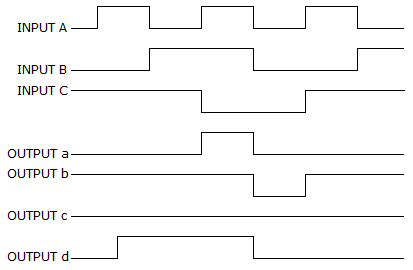# Digital Electronics - Describing Logic Circuits

## Why Digital Electronics Describing Logic Circuits?

In this section you can learn and practice Digital Electronics Questions based on "Describing Logic Circuits" and improve your skills in order to face the interview, competitive examination and various entrance test (CAT, GATE, GRE, MAT, Bank Exam, Railway Exam etc.) with full confidence.

## Where can I get Digital Electronics Describing Logic Circuits questions and answers with explanation?

IndiaBIX provides you lots of fully solved Digital Electronics (Describing Logic Circuits) questions and answers with Explanation. Solved examples with detailed answer description, explanation are given and it would be easy to understand. All students, freshers can download Digital Electronics Describing Logic Circuits quiz questions with answers as PDF files and eBooks.

## Where can I get Digital Electronics Describing Logic Circuits Interview Questions and Answers (objective type, multiple choice)?

Here you can find objective type Digital Electronics Describing Logic Circuits questions and answers for interview and entrance examination. Multiple choice and true or false type questions are also provided.

## How to solve Digital Electronics Describing Logic Circuits problems?

You can easily solve all kind of Digital Electronics questions based on Describing Logic Circuits by practicing the objective type exercises given below, also get shortcut methods to solve Digital Electronics Describing Logic Circuits problems.

### Exercise :: Describing Logic Circuits - General Questions

1.

The format used to present the logic output for the various combinations of logic inputs to a gate is called a(n):

 A. truth table. B. input logic function. C. Boolean constant. D. Boolean variable.

Explanation:

No answer description available for this question. Let us discuss.

2.

What is the basic difference between AHDL and VHDL?

 A. ADHL is used in all PLD's. B. VHDL is used in all PLD's. C. ADHL is proprietary. D. VHDL is proprietary.

Explanation:

No answer description available for this question. Let us discuss.

3.

A small circle on the output of a logic gate is used to represent the:

 A. Comparator operation. B. OR operation. C. NOT operation. D. AND operation.

Explanation:

No answer description available for this question. Let us discuss.

4.

For a three-input OR gate, with the input waveforms as shown below, which output waveform is correct?A. a B. b C. c D. d

Explanation:

No answer description available for this question. Let us discuss.

5.

Which of the figures given below represents a NOR gate?A. a B. b C. c D. d